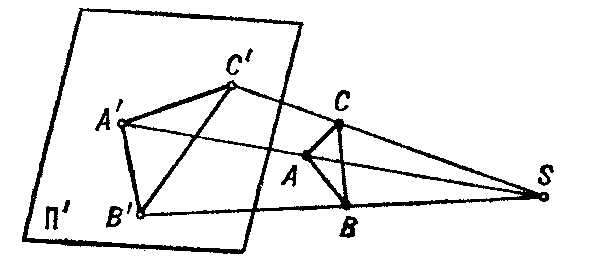# Projection

(diff) ← Older revision | Latest revision (diff) | Newer revision → (diff)

A term related to the operation of projecting, which can be defined as follows (see Fig.): One chooses an arbitrary point $S$ of the space as the centre of projection and a plane $\Pi ^ \prime$ not passing through $S$ as the plane of projection. To project a point $A$( a pre-image) of the space onto the plane $\Pi ^ \prime$ through the centre $S$, one draws the straight line $S A$ to its intersection with the plane $\Pi ^ \prime$ at a point $A ^ \prime$. The point $A ^ \prime$( the image) is called the projection of $A$. The projection of a figure $F$ is defined to be the collection of projections of all its points.Figure: p075130a

The projection just described is called central (or conical). A projection with centre of projection at infinity is called parallel (or cylindrical). If, moreover, the plane of projection is perpendicular to the direction of projection, then the projection is called orthogonal.

Parallel projections are widely used in descriptive geometry for obtaining various types of images (see, for example, Axonometry; Perspective). There are special forms of projections onto the plane, sphere and other surfaces (see, for example, Cartographic projection; Stereographic projection).

In geometry and linear algebra one also encounters projections parallel to a subspace. For instance, if $X$ is a vector space, $V$ a subspace and $W$ a complementary subspace (i.e. $V \cap W = \{ 0 \}$ and $X = V+ W$), then the projection $P$ from $X$ onto $V$ parallel to $W$ is the linear mapping that sends $x = v+ w$, $v \in V$, $w \in W$, to $v$. The operator $P$ satisfies $P ^ {2} = P$, and each such operator comes from a decomposition $X = V \oplus W$ with $V = P( X)$, $W = ( I- P)( X)$.
The orthogonal projection of a Hilbert space $H$ to a closed subspace $F$ assigns to $x \in H$ the unique element $y$ of $F$ such that $x - y$ and $F$ are orthogonal. It is the parallel projection onto $F$ along the orthogonal complement $F ^ \perp = \{ {x \in H } : {\langle x, y \rangle = 0 \textrm{ for all } y \in F } \}$. The element $y$ is the element of best approximation to $x$ in $F$. In this case the corresponding operator $P$ is also self-adjoint, and, conversely, self-adjoint operators $P$ such that $P ^ {2} = P$ are orthogonal projections. Cf. also Projector.